• Mood:

# Oracle's Database

 Taken Characters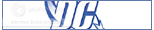S u p e r m a n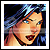B a t m a n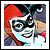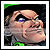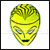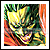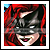T e e n T i t a n s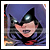T h e O u t s i d e r sG r e e n L a n t e r n C o r p sW o n d e r W o m a nG e n 1 3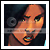V f o r V e n d e t t a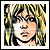T h e M a x x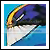F a b l e s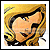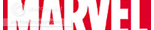T h e P h o e n i x F o r c e a n d J e a n G r e yX - m e n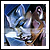S p i d e r - M a nI r o n M a n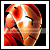T h e P u n i s h e rD a r e d e v i lD e a d p o o l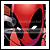A s s o r t e d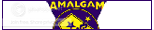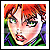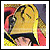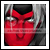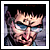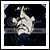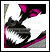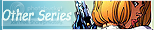T h e D a r k n e s sN e w s A n c h o r s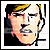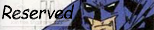*n o n e c u r r e n t l y*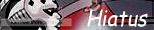*n o n e c u r r e n t l y*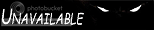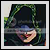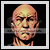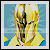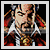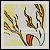[Main Community] [OOC] [Application] [FAQ] [Character Wishlist]

• #### Post Format

Post Format Who:(What character is posting) What:(One line describing post content) When:(What time of day is it?) Where:(Where is…

• #### Contact Q!

OOC Journal: AIM: AnarchicQ YIM: N/A Email/MSN: AnarchicQ AT gmail dot com Characters: - Deadpool - The…

• #### MDC FAQ

FAQ So this is one of those "World Mergy" thingies. How do our memories work? Aside from characters that are insane enough to have 4th…

Comments for this post were disabled by the author